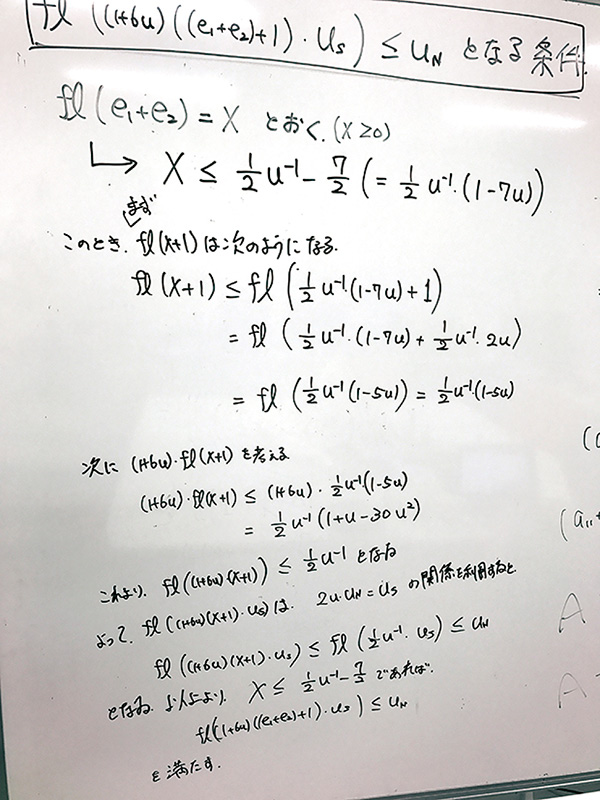# Numerical Analysis Laboratory

## Creating a world where calculation results can be trusted

You think PCs are a convenient machine capable of making calculations accurately and speedily, don’t you? But they are only capable of calculating speedily only if the numerals, whose number of digits is finite and which are expressed with binary numbers, are used. Even if they have a finite number of digits, errors occur in calculations, which sometimes result in grossly inaccurate answers. Our laboratory closely examines these errors to develop methods for practical use to obtain reliable results.Affiliation Mathematical Sciences Faculty Name OZAKI, Katsuhisa Academic Society The Japan Society for Industrial and Applied MathematicsJapan Society for Simulation TechnologyInformation Processing Society of Japan Keyword Verified Numerical Computations, Computer Assisted Proof, High Performance Computing, Interval Analysis

### Study Fields

• Numerical analyses
• Information science
• Mathematics
• Applied mathematics

### For Society

The results of our research will contribute mainly to information-related fields, such as improving simulation technologies, by enhancing the precision of numerical calculations, cutting costs by making the calculation speed faster and preventing simulation algorithm failures.

### Research Themes

• Research on numerical calculations with precision guarantee of problems using matrix
• Development of high-speed and robust algorithms in computational geometry
• Research on efficient parallel programing in multi-core processing